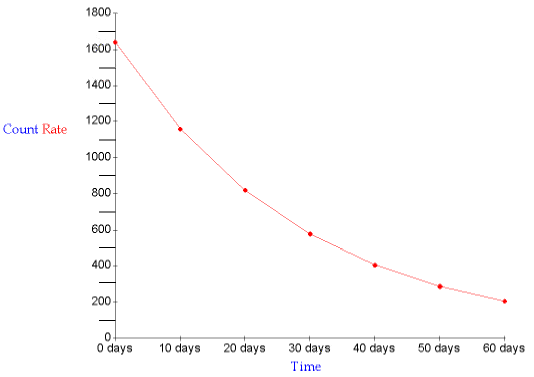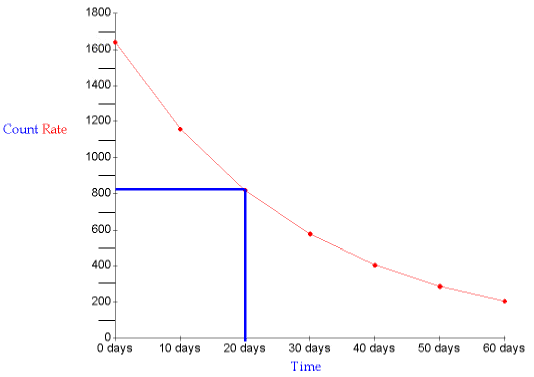gcsescience.com                                       17                                       gcsescience.com

Measuring Half-life from a Graph.

How can Half-life be Measured from a Graph?

A graph showing how the count rate decreases as time goes by
will have a curve like the one shown in the picture below.
For any particular radioisotope the count rate and time will
be different but the shape of the curve will be the same.The easiest way to measure the half-life from the graph is to

1. Read the original count rate at zero days.
On our graph the reading is 1640 counts.

2. Go down to half the original count rate (820 counts)
and draw a horizontal line to the curve.
Then draw a vertical line down from the curve.
You can read off the half-life where the line crosses the time axis.On our graph the half-life is 20 days.

gcsescience.com     Physics Quiz     Index     Radioactivity Quiz     gcsescience.com